QRコード(所蔵情報)### Differential equations and mathematical physics : proceedings of the international conference held at the University of Alabama at Birmingham, March 15-21, 1990

フォーマット:

edited by Christer Bennewitz

Boston, Mass. : Academic Press, c1992

xxx, 365 p. ; 24 cm

シリーズ名:
Mathematics in science and engineering : a series of monographs and textbooks ; v. 186 <BA00009880>

BA12744519
ISBN:
9780120890408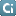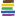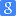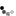タイトル・著者・出版者が同じ資料

### 類似資料:

1

2

3

4

5

6

7

8

9

10

11

12

 1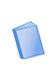図書 Differential equations and mathematical physics : proceedings of an international conference held at the University of Alabama in Birmingham, March 16-20, 1999 Weikard, Rudi, Weinstein, Gilbert, International Conference on Differential Equations and Mathematical Physics American Mathematical Society, International Press 7図書 Numerical approximation of partial differential equations : selection of papers presented at the International Symposium on Numerical Analysis, held at the … International Symposium on Numerical Analysis, Ortiz, Eduardo L. North-Holland 2図書 Differential equations : proceedings of the conference held at the University of Alabama in Birmingham, Birmingham, Alabama, U.S.A., 21-26 March, 1983 Lewis, Roger T., 1942-, International Conference on Differential Equations, Knowles, Ian W., 1946- North-Holland, Sole distributors for the U.S.A. and Canada, Elsevier Science Pub. Co. 8図書 Conference on the Numerical Solution of Differential Equations : held in Dundee/Scotland, June 23-27, 1969 Conference on the Numerical Solution of Differential Equations, Morris, John Ll. Springer-Verlag 3図書 Advances in differential equations and mathematical physics : UAB International Conference, Differential Equations and Mathematical Physics, March 26-30, 2002, … International Conference on Differential Equations and Mathematical Physics, Karpeshina, Yulia E., 1956- American Mathematical Society 9図書 Numerical methods for ordinary differential equations : proceedings of the workshop held in L'Aquila (Italy), Sept. 16-18, 1987 Bellen, A. (Alfredo), 1941-, Gear, Charles William, 1935-, Russo, E. (Elvira), 1940-, Symposium on Ordinary Differential … Springer-Verlag 4図書 Differential equations and mathematical physics : proceedings of the conference sponsored by University of Alabama at Birmingham and Georgia Institute of … Knowles, Ian International Press 10図書 Differential equations : stability and control Elaydi, Saber, 1943-, International Conference on Differential Equations: Theory and Applications in Stability and … M. Dekker 5図書 Recent advances in differential equations and mathematical physics : UAB International Conference on Differential Equations and Mathematical Physics, March … Chernov, Nikolai, 1956-, Karpeshina, Yulia E., 1956-, Knowles, Ian W., 1946-, Lewis, Roger T., 1942-, Weikard, Rudi, … American Mathematical Society 11図書 Differential geometric methods in mathematical physics : proceedings of an international conference held at the Technical University of Clausthal, FRG, August … Hennig, Jörg Dieter, Doebner, Heinz Dietrich, 1931- Springer-Verlag 6図書 Numerical solutions of boundary value problems for ordinary differential equations : [proceedings of a symposium held at the University of Maryland, Baltimore … Symposium on Numerical Solutions of Boundary Value Problems for Ordinary Differential Equations, Aziz, Abdul Kadir, … Academic Press 12図書 Geometric methods in mathematical physics : proceedings of an NSF-CBMS conference held at the University of Lowell, Massachusetts, March 19-23, 1979 Kaiser, Gerald, 1940-, Marsden, Jerrold E., 1942-, National Science Foundation (U.S.), Conference Board of the … Springer-Verlag